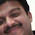## Tuesday, July 5, 2011

### Finding the Index of a lens

Using the Thin Lens Theory you can calculate the Index Of the Material given !

just find out the Back Vertex Power of the Lens with the help of Focimeter & ADD the surface powers together to find the THIN LENS POWER.
NOW ,The Ratio of the thin lens power to the true back vertex power is the same value as the CVF ( CURVE VARIATION FACTOR ) of the Material of the LENS.

Formula 1:
nT=1+Ft(nLM-1)/Flm

where Ft = true back vertex power of the lens
Flm = sum of the both surface powers ( Thin Lens Power )
nLM = refractive index for which the lens measure has been scaled
nT = REFRACTIVE INDEX OF THE MATERIAL OF THE LENS .

Simplified as;

Formula 2:
n=(0.53 x Fv/Fc )+1 (great and simplified formula for spheric lenses)

where n is the refractive index, FV is the back vertex power of the lens as measured with a focimeter and FC is the power of the lens as measured with a lens clock by adding the front and back surface measurements together. This assumes that the lens clock is calibrated for the standard tooling index of 1.53.

eg:

if back vertex power is -6.00d, -4.75 is the power of the lens as measured with lens clock by adding the front and back surface measurements then the index of the material will be,

n=(0.53 x -6.00/-4.75) + 1
n=(0.53x1.2632) + 1
n= 0.6694 + 1
n= 1.67

1.pls tell how to find Fv accurately

2.hi Salil
Fv is the back vertex power which you can easily measured with a lens meter. (eg: -5.00sph, +3.50sph), then calculate the accurate front curvature and back curvature add the values. TThis value will be very near to Fv.

for eg: I have -5.00sph 1.50 lens

so Fv will be -5.00
front curvature i have measured with lens clock is +1.83
also the back curvature is -7.00

then this formula can write with the values as below;
n=(0.53x(-5.00/(+1.83-7.00))+1
n=(0.53x(-5.00/-5.17))+1
n=(0.53x0.97)+1
n=0.51+1
n=1.51

so that the lens index is 1.50

3.hi tell me the index of a lens if the power is -3.50 and the front curvature is +3.50 and back curvature is -7.00

first of all let me calculate what happen.
n=(0.53x(-7.00/(+3.50-7.00)+1
n=(0.53x(-7.00/-3.50)+1
n=(0.53x2)+1
n=1.06+1
n=2.06

here i would like to say the index of lens which has been given to me is 1.56

so please tell me how reliable this formula.

4.Your calculation have a slight error. It should be as below:
n=(0.53x(-3.50/(+3.5-7)))+1
n=(0.53x(-3.50/-3.50))+1
n=(0.53x(1))+1
n=0.53+1
n=1.53
So if your measurements are correct, the refractive index will be 1.53

5.ok thanks

now how can we measure toric lens index

6.In the case of toric, you can use the same formula with a little care.
just mark 180 degree and follow the sph power and measure the lens clock on the axis.

1.can you give an example please??

7.A lens has power of +4.0D in air, and +1.73D in water (n=1.33). What’s the index of the lens? index of the lens?